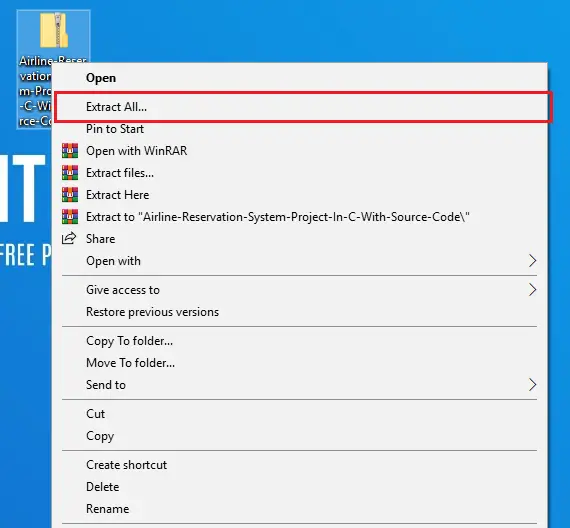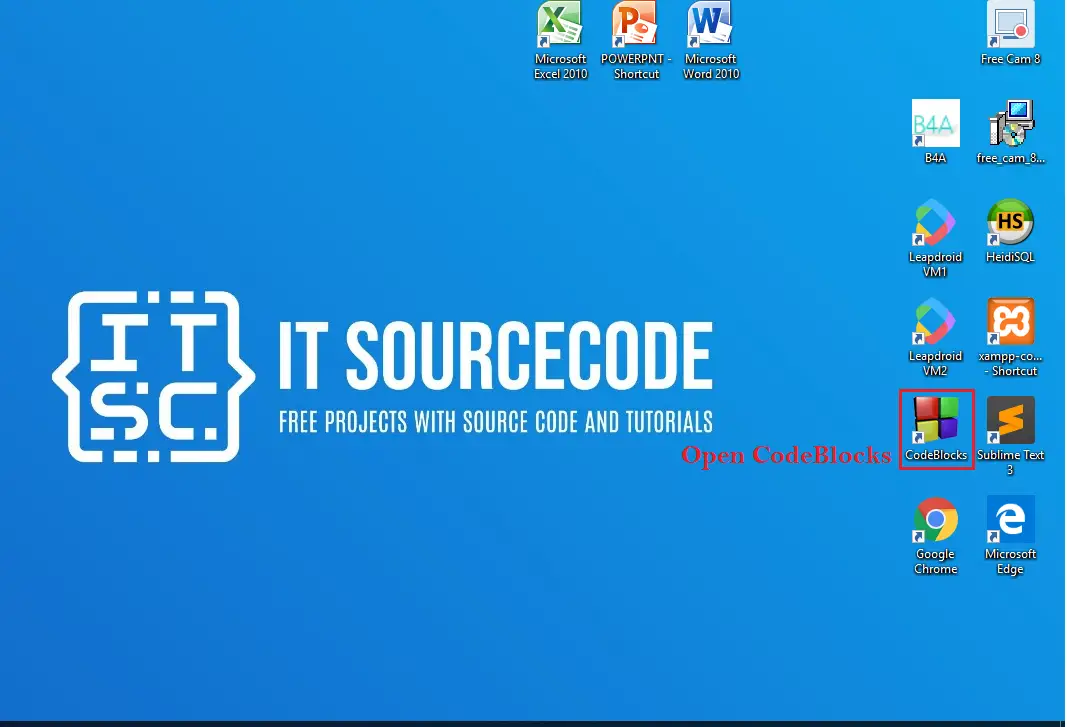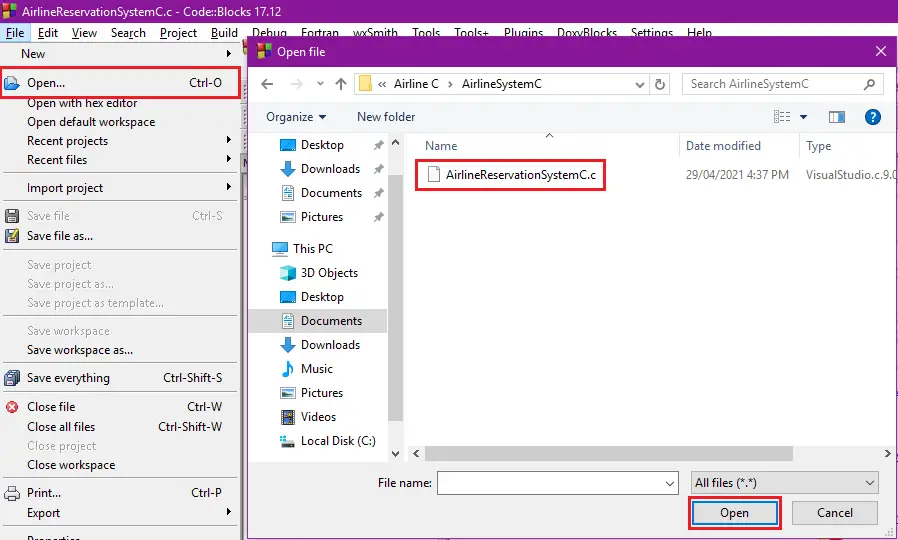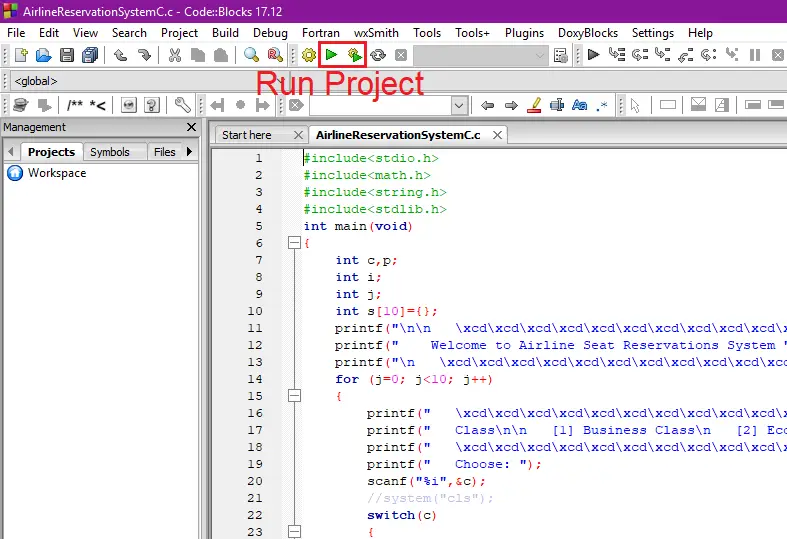# Airline Reservation System Project In C With Source Code

## Airline Reservation System Project In C With Source Code

The Airline Reservation System Project In C With Source Code is developed using C programming language. This airline reservation system in c source code is a console application that can let you assign a reservation seat.

A simple airline reservation system in c programming requires the user to choose between Business and Economy class seats. This project then shows available seats, and the user must enter the seat number to reserve the particular seat. After you have reserved a seat, it will no longer be open for other users.

This airline reservation system in c mini project is a console application project with a simple coding structure that beginners can easily understand and learn.

Airline Reservation System Project ...
Airline Reservation System Project in Java and MySQL with Source Code (Free Download) 2022

Anyway if you want level up your knowledge in programming especially C/C++ Programming Language, try this new article I’ve made for you Best C Projects with Source Code for Beginners Free Download 2022.

To run this airline reservation system in c make sure that you have a Code Blocks or any platform of C installed in your computer.

## Airline Reservation System Project In C With Source CodeSteps On How To Run The Project

Time needed: 5 minutes.

These are the steps on how to run Airline Reservation System Project In C With Source Code• Step 2: Extract file.

Second, after you finished download the source code, extract the zip file.• Step 3: Open CodeBlocks

Third, open “CodeBlocks IDE”.• Step 4: Open Project.

Fourth, open file tab and Open File after that open folder AirlineSystemC then click the “AirlineReservationSystemC.c“.• Step 5: Run Project

Fifth, run the project.• Step 6: The actual code.

#### The Code Given Below is the Actual Code of System

```#include<stdio.h>
#include<math.h>
#include<string.h>
#include<stdlib.h>
int main(void)
{
int c,p;
int i;
int j;
int s={};
printf("\n\n   \xcd\xcd\xcd\xcd\xcd\xcd\xcd\xcd\xcd\xcd\xcd\xcd\xcd\xcd\xcd\xcd\xcd\xcd\xcd\xcd\xcd\xcd\xcd\xcd\xcd\xcd\xcd\xcd\xcd\xcd\xcd\xcd\xcd\xcd\xcd\xcd\xcd\xcd\xcd\xcd\xcd\xcd\xcd\xcd\xcd\n");
printf("    Welcome to Airline Seat Reservations System ");
printf("\n   \xcd\xcd\xcd\xcd\xcd\xcd\xcd\xcd\xcd\xcd\xcd\xcd\xcd\xcd\xcd\xcd\xcd\xcd\xcd\xcd\xcd\xcd\xcd\xcd\xcd\xcd\xcd\xcd\xcd\xcd\xcd\xcd\xcd\xcd\xcd\xcd\xcd\xcd\xcd\xcd\xcd\xcd\xcd\xcd\xcd\n\n");
for (j=0; j<10; j++)
{
printf("   \xcd\xcd\xcd\xcd\xcd\xcd\xcd\xcd\xcd\xcd\xcd\xcd\xcd\xcd\xcd\xcd\xcd\xcd\xcd\xcd\xcd\xcd\xcd\xcd\xcd\xcd\xcd\xcd\xcd\xcd\xcd\xcd\xcd\xcd\xcd\xcd\xcd\xcd\xcd\xcd\xcd\xcd\xcd\xcd\xcd\n");
printf("   Class\n\n    Business Class\n    Economic class\n\n");
printf("   \xcd\xcd\xcd\xcd\xcd\xcd\xcd\xcd\xcd\xcd\xcd\xcd\xcd\xcd\xcd\xcd\xcd\xcd\xcd\xcd\xcd\xcd\xcd\xcd\xcd\xcd\xcd\xcd\xcd\xcd\xcd\xcd\xcd\xcd\xcd\xcd\xcd\xcd\xcd\xcd\xcd\xcd\xcd\xcd\xcd\n");
printf("   Choose: ");
scanf("%i",&c);
//system("cls");
switch(c)
{
case 1:
printf("   \xcd\xcd\xcd\xcd\xcd\xcd\xcd\xcd\xcd\xcd\xcd\xcd\xcd\xcd\xcd\xcd\xcd\xcd\xcd\xcd\xcd\xcd\xcd\xcd\xcd\xcd\xcd\xcd\xcd\xcd\xcd\xcd\xcd\xcd\xcd\xcd\xcd\xcd\xcd\xcd\xcd\xcd\xcd\xcd\xcd\n");
printf("   Seats available\n     |1|2|3|4|5|\n\n");
printf("   \xcd\xcd\xcd\xcd\xcd\xcd\xcd\xcd\xcd\xcd\xcd\xcd\xcd\xcd\xcd\xcd\xcd\xcd\xcd\xcd\xcd\xcd\xcd\xcd\xcd\xcd\xcd\xcd\xcd\xcd\xcd\xcd\xcd\xcd\xcd\xcd\xcd\xcd\xcd\xcd\xcd\xcd\xcd\xcd\xcd\n");
do
{
printf("   Pick a seat: ");
scanf("%i",&p);
s[j]=p;
//system("cls");
for (i=0; i<j; i++)
{
if (s[j]==s[i])
{
printf("\n\n   Seat taken.\n\n");
break;
}
}
}
while (i!=j);
if(s[j] <= 5)
{
printf("   \xcd\xcd\xcd\xcd\xcd\xcd\xcd\xcd\xcd\xcd\xcd\xcd\xcd\xcd\xcd\xcd\xcd\xcd\xcd\xcd\xcd\xcd\xcd\xcd\xcd\xcd\xcd\xcd\xcd\xcd\xcd\xcd\xcd\xcd\xcd\xcd\xcd\xcd\xcd\xcd\xcd\xcd\xcd\xcd\xcd\n");
printf("\n");
printf("   Seat no : %i\n",s[j]);
printf("\n");
}
else
printf("\nWrong number!  No seat for you!\n\n");
break;
case 2:
printf("   \xcd\xcd\xcd\xcd\xcd\xcd\xcd\xcd\xcd\xcd\xcd\xcd\xcd\xcd\xcd\xcd\xcd\xcd\xcd\xcd\xcd\xcd\xcd\xcd\xcd\xcd\xcd\xcd\xcd\xcd\xcd\xcd\xcd\xcd\xcd\xcd\xcd\xcd\xcd\xcd\xcd\xcd\xcd\xcd\xcd\n");
printf("   Economical class\n\n");
printf("   Seats available\n    |6|7|8|9|10|\n\n");
printf("   \xcd\xcd\xcd\xcd\xcd\xcd\xcd\xcd\xcd\xcd\xcd\xcd\xcd\xcd\xcd\xcd\xcd\xcd\xcd\xcd\xcd\xcd\xcd\xcd\xcd\xcd\xcd\xcd\xcd\xcd\xcd\xcd\xcd\xcd\xcd\xcd\xcd\xcd\xcd\xcd\xcd\xcd\xcd\xcd\xcd\n");
do
{
printf("   Pick a seat: ");
scanf("%i",&p);
s[j]=p;
//system("cls");
for (i=0; i<j; i++)
{
if (s[j]==s[i])
{
printf("\n\n   Seat taken.\n\n");
break;
}
}
}
while (i!=j);
if(s[j] >= 6)
{
printf("   \xcd\xcd\xcd\xcd\xcd\xcd\xcd\xcd\xcd\xcd\xcd\xcd\xcd\xcd\xcd\xcd\xcd\xcd\xcd\xcd\xcd\xcd\xcd\xcd\xcd\xcd\xcd\xcd\xcd\xcd\xcd\xcd\xcd\xcd\xcd\xcd\xcd\xcd\xcd\xcd\xcd\xcd\xcd\xcd\xcd\n");
printf("\n");
printf("   Class: Economical class\n");
printf("   Seat no : %i\n",s[j]);
printf("\n");
}
else
printf("\nWrong number!  No seat for you!\n\n");
break;
default:
break;
}//end switch case
}//end counting people*/
return 0;
}
```